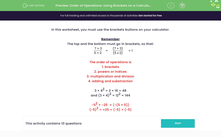# Order of Operations: Using Brackets on a Calculator

In this worksheet, students use the order of operations and the brackets buttons on a calculator to arrive at answers to problems.Key stage:  KS 3

Curriculum topic:   Number

Curriculum subtopic:   Use Calculators/Technology for Accuracy

Difficulty level:#### Worksheet Overview

In this worksheet, you must use the brackets buttons on your calculator.

Remember

The top and the bottom must go in brackets, so that:

 7 + 3 5 × 2
=
 (7 + 3) (5 x 2)
= 1

The order of operations is

1. brackets

2. powers or indices

3. multiplication and division

3 × 42 = 3 × 16 = 48

and (3 × 4)2 = 122 = 144

−52 = −25  = (−(5 × 5))

(−5)2 = +25 = (−5) × (−5)

### What is EdPlace?

We're your National Curriculum aligned online education content provider helping each child succeed in English, maths and science from year 1 to GCSE. With an EdPlace account you’ll be able to track and measure progress, helping each child achieve their best. We build confidence and attainment by personalising each child’s learning at a level that suits them.

Get started